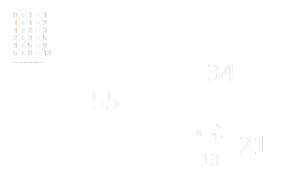# Fibonacci Sequence of NumbersComputations:
Sequence:

Displays the Fibonacci Sequence of Numbers as entered by the user. Warning: This is very processor intensive, do not enter a large number.

## What are the numbers in the Fibonacci sequence?

The Fibonacci sequence is a sequence of integers in which each term is the sum of the two terms that precede it. It owes its name to Leonardo Fibonacci, an Italian mathematician. The golden ratio seems to be very present in nature in multiple forms, in plants: phyllotaxis (arrangement of leaves), the geometry of flowers ... but also in architecture and in the arts.

## How to find the Fibonacci sequence?

In the Fibonacci sequence the Fibonacci sequence, it is not necessary to memorize each of the terms or numbers of the sequence (which is by the way infinite). It is enough to remember its rule of construction: with the exception of the first two, each term of the sequence is equal to the sum of the two terms which immediately precede it, in other words, it is a question of a sequence of numbers in which any number (starting from the third) is equal to the sum of the previous two:

1, 1, 2, 3, 5, 8, 13, 21, 34, 55, 89, ...

Just take two starting numbers. Adding them gives the third, then the second + the third gives the fourth, and so on. The terms of this sequence are called Fibonacci numbers.

The Fibonacci sequence has many properties that are widely used in mathematics. One of them is that the ratio of two consecutive numbers in the sequence is alternately greater and less than the golden ratio, a remarkable number that is equal to exactly 1.61803398 ...

## Are Fibonacci numbers prime?

In arithmetic, a prime Fibonacci number is a Fibonacci number which is also prime

The seven smallest Fibonacci primes Fn are 2, 3, 5, 13, 89, 233, and 1,597, and the corresponding n indices are 3, 4, 5, 7, 11, 13, and 17.

We do not know if there is an infinity of prime Fibonacci numbers. We know that Fn divides Fkn , and therefore that, for all n> 4, if Fn is prime, then n is prime, but the converse is false (F19= 4181 = 37x113 is the first counterexample). As of October 2015, the greatest known prime Fibonacci number is F81839 and the largest known possibly prime Fibonacci number is F2904353.